﻿ 多波束声呐基阵一体化自校准方法
«上一篇文章快速检索 高级检索

 哈尔滨工程大学学报2019, Vol. 40Issue (4): 792-798  DOI: 10.11990/jheu.2018060490

### 引用本文WEI Bo, ZHOU Tian, LI Chao, et al. Research on the self-calibration of multi-beam echo sounder transducer array based on the integrated method[J]. Journal of Harbin Engineering University, 2019, 40(4), 792-798. DOI: 10.11990/jheu.201806049.### 文章历史

1. 哈尔滨工程大学 水声技术重点实验室, 黑龙江 哈尔滨 150001;
2. 哈尔滨工程大学, 海洋信息获取与安全工信部重点实验室, 黑龙江 哈尔滨 150001;
3. 哈尔滨工程大学 水声工程学院, 黑龙江 哈尔滨 150001

Research on the self-calibration of multi-beam echo sounder transducer array based on the integrated method
WEI Bo 1,2,3, ZHOU Tian 1,2,3, LI Chao 1,2,3, XING Tianyao 1,2,3, LI Haisen 1,2,31. Acoustic Science and Technology Laboratory, Harbin Engineering University, Harbin 150001, China;
2. Key Laboratory of Marine Information Acquisition and Security, Harbin Engineering University, Harbin 150001, China;
3. College of Underwater Acoustic Engineering, Harbin Engineering University, Harbin 150001, China
Abstract: To ensure detection precision of the multi-beam detection system, the directivity of the multi-element transducer array needs to be measured and the beam response in the object direction needs to be calibrated before the system is assembled.The conventional array directivity measurement methods usually assume that the received signal fits the far-field plane wave model.In this paper, we analyze the defocus problem caused by far-field approximation and deduce the depth error caused by the element spacing error.We propose a novel self-calibration method for multi-beam echo sounder (MBES) transducer array that is based on the method of focused beamforming to overcome the deficiencies of conventional method.An experiment was carried out in a small-size tank, and the field experimental data was compensated by the beam angle error curve obtained in the tank experiment.The experimental results demonstrated that the proposed self-calibration approach can guarantee the coverage of the MBES system; thus, the method has strong practicability and high engineering value and can therefore be promoted.
Keywords: transducer array calibration    near-field focused beamforming    multi-beam echo sounder    image defocus

1 基础理论 1.1 换能器基阵信号模型

 ${\tau _0} = \frac{d}{c}\sin \theta$ (1)

 $\mathit{\boldsymbol{w}}\left( \theta \right) = {\left[ {\begin{array}{*{20}{c}} 1&{{{\rm{e}}^{ - {\rm{j}}\omega {\tau _0}}} \cdots {{\rm{e}}^{ - {\rm{j}}\omega \left( {M - 1} \right){\tau _0}}}} \end{array}} \right]^{\rm{T}}}$ (2)

 $\mathit{\boldsymbol{R}} = \frac{1}{N}\sum\limits_{t = 1}^N {\mathit{\boldsymbol{x}}\left( t \right){\mathit{\boldsymbol{x}}^{\rm{H}}}\left( t \right)}$ (3)

 ${P_{{\rm{CBF}}}}\left( \theta \right) = {\mathit{\boldsymbol{w}}^{\rm{H}}}\left( \theta \right)\mathit{\boldsymbol{Rw}}\left( \theta \right)$ (4)

 $\begin{array}{*{20}{c}} {{P_{{\rm{CBF}}}}\left( \theta \right) = \frac{1}{N}\sum\limits_{t = 1}^N {{{\left| {y\left( {t,\theta } \right)} \right|}^2}} = }\\ {\frac{1}{N}\sum\limits_{t = 1}^N {{{\left| {\sum\limits_{i = 1}^M {{{\rm{e}}^{ - {\rm{j}}\left( {i - 1} \right)\omega {\tau _0}}}} } \right|}^2}} } \end{array}$ (5)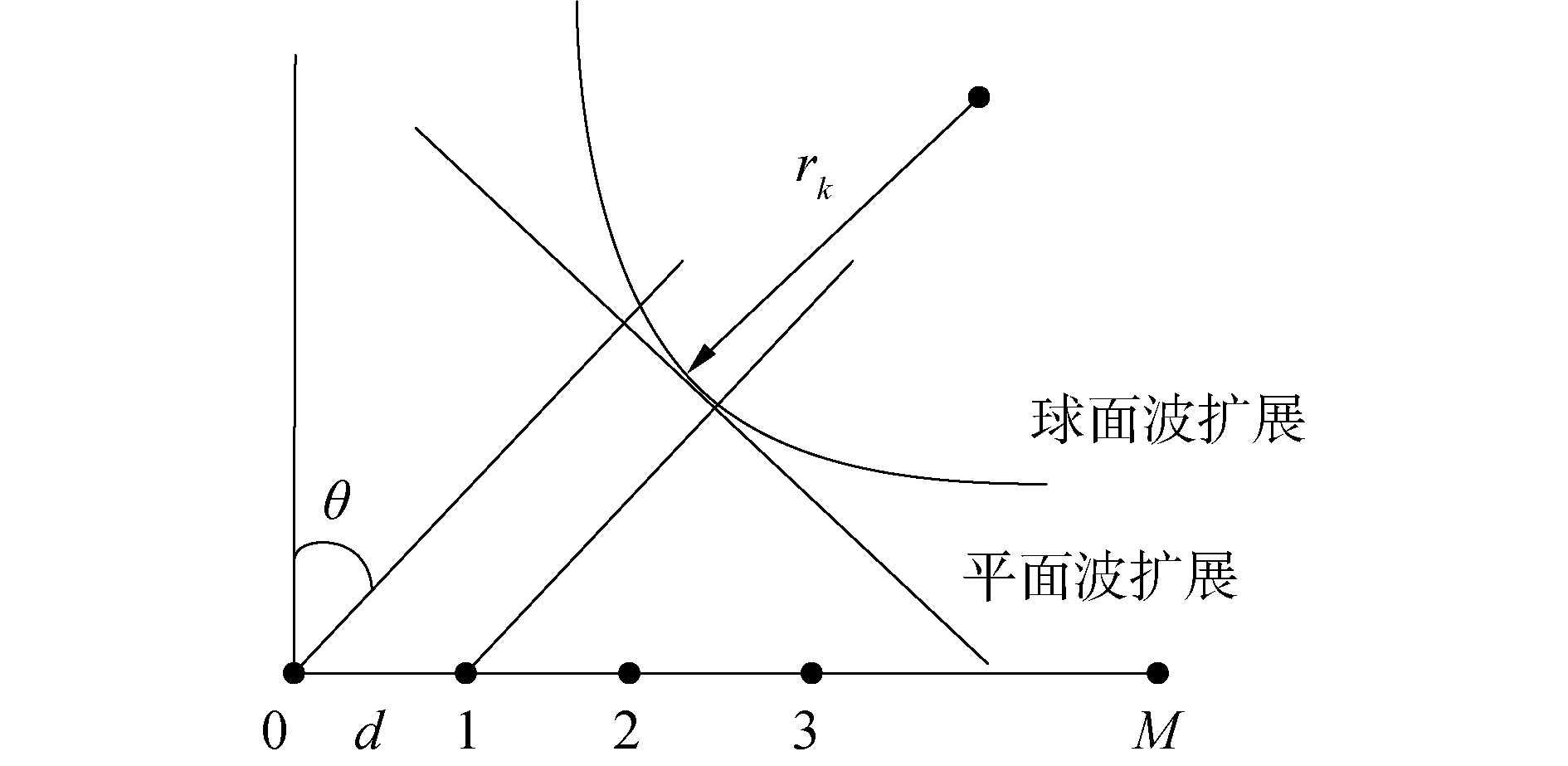Download: 图 1 多波束基阵回波到达模型 Fig. 1 Echo model of the multibeam transducer array

 ${\varphi _{mk}} \approx - 2{\rm{ \mathsf{ π} }}\frac{{md}}{\lambda }\sin {\theta _k} + {m^2}{\rm{ \mathsf{ π} }}\frac{{{d^2}}}{{\lambda {r_k}}}{\cos ^2}{\theta _k}$ (6)

 $V\left( {{\theta _k}} \right) = \sum\limits_{m = 0}^{M - 1} {{V_m}\left( {\cos {\varphi _{mk}} - {\rm{j}}\sin {\varphi _{mk}}} \right)}$ (7)
1.2 近场聚焦成像仿真表 1 目标仿真参数 Table 1 Target simulation parameters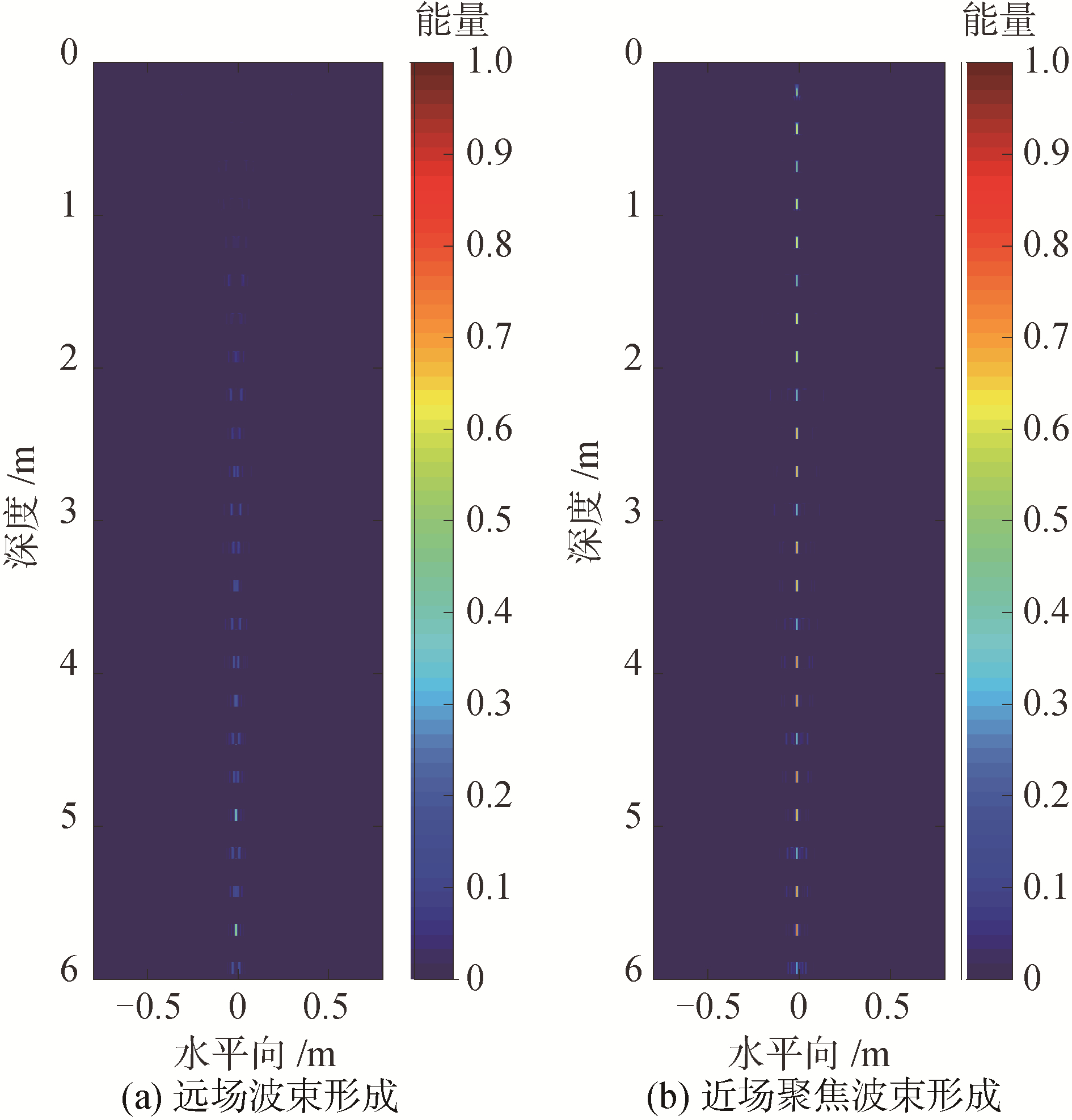Download: 图 2 远场波束形成与近场聚焦波束形成输出对比 Fig. 2 Comparison of the far-field beamforming and the near-filed focused beamforming
2 基阵误差分析 2.1 阵元间距误差对DOA估计的影响

 $\sin \theta = \frac{{\beta \lambda }}{{2{\rm{ \mathsf{ π} }}d}}$ (8)

 ${\mathop{\rm si}\nolimits} n'\theta = \frac{{\beta \lambda }}{{2{\rm{ \mathsf{ π} }}\left( {d + \Delta d} \right)}}$ (9)

 $\Delta \theta = \arcsin \frac{{\beta \lambda }}{{2{\rm{ \mathsf{ π} }}d}} - \arcsin \frac{{\beta \lambda }}{{2{\rm{ \mathsf{ π} }}\left( {d + \Delta d} \right)}}$ (10)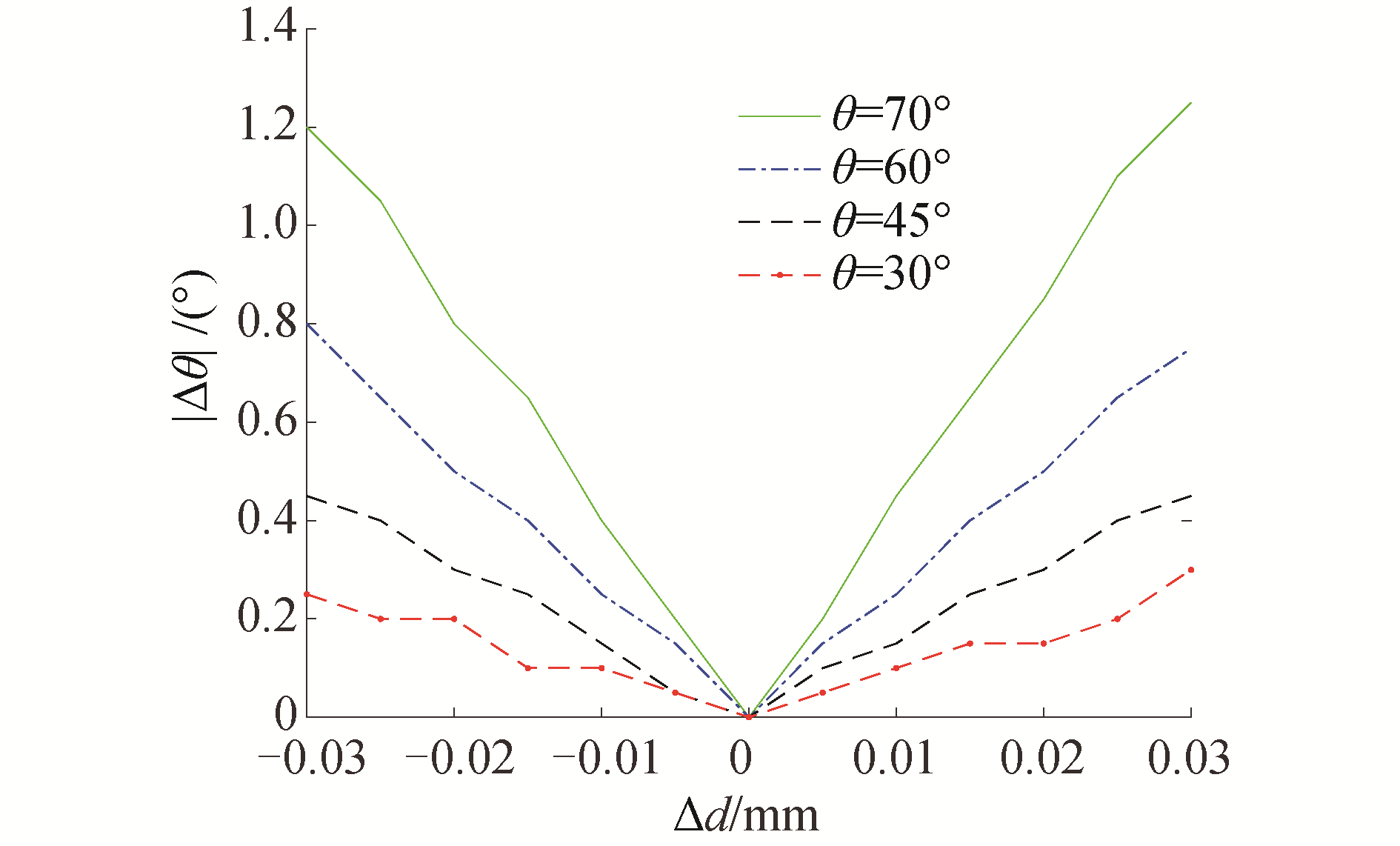Download: 图 3 波束到达方向误差曲线 Fig. 3 Error curves of the direction of arrival
2.2 DOA估计误差对测深精度产生的影响

 $H' = \frac{H}{{\cos \theta }} \cdot \cos \left( {\theta + \Delta \theta } \right)$ (11)

 $\Delta H' = H\left( {1 - \frac{{\cos \left( {\theta + \Delta \theta } \right)}}{{\cos \theta }}} \right)$ (12)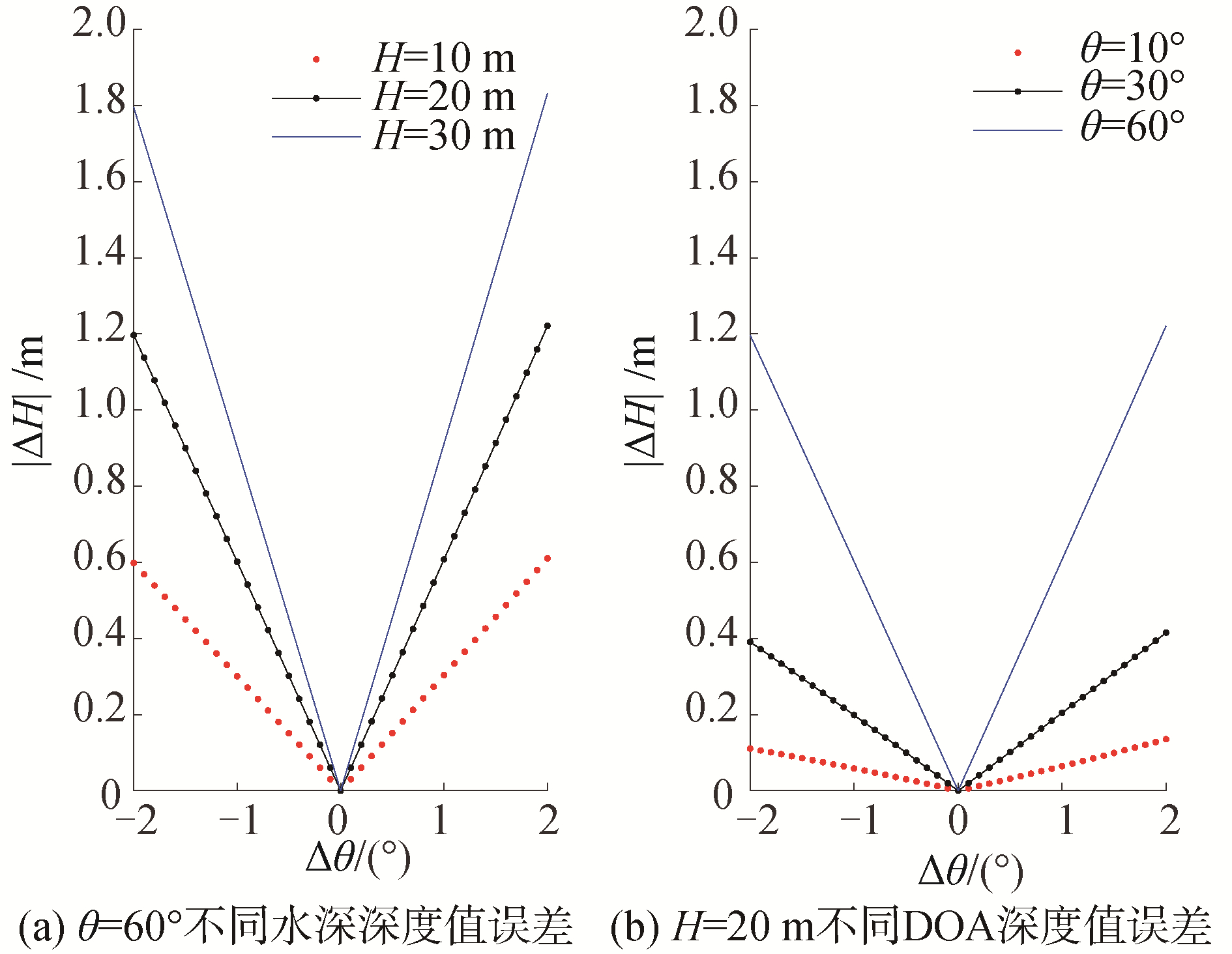Download: 图 4 不同条件下深度测量误差曲线 Fig. 4 Depth error curve at different parameters

3 基阵一体化自校准方法研究 3.1 机械结构设定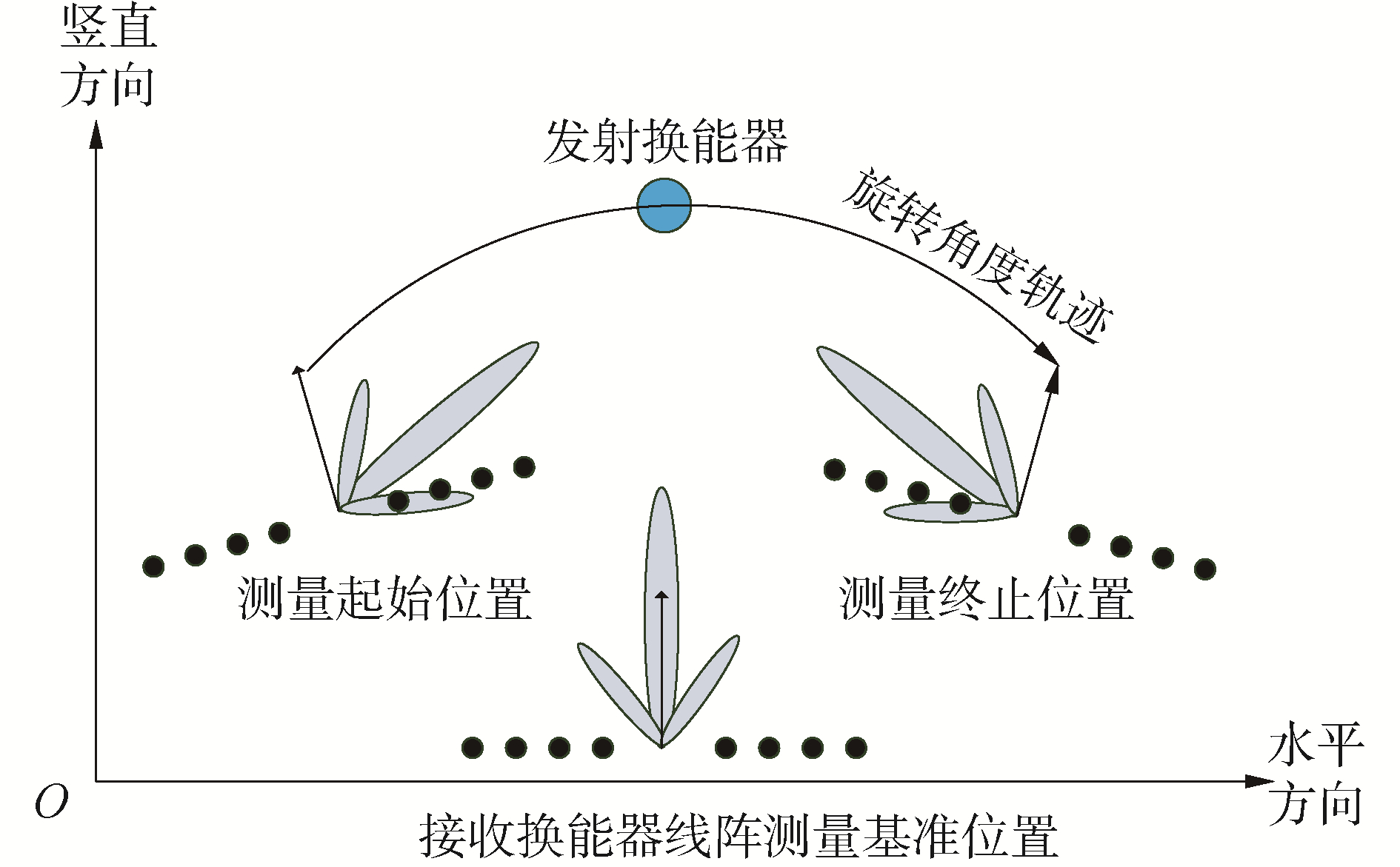Download: 图 5 基阵旋转及波束指向性示意 Fig. 5 Rotating transducer array and the beam directivity

3.2 信号处理流程

 ${K_i} = L/\cos {\theta _i}$ (13)

 ${N_i} \in \left[ {\left( {1 - \alpha } \right)\frac{{2{K_i}}}{{c{f_s}}},\left( {1 + \alpha } \right)\frac{{2{K_i}}}{{c{f_s}}}} \right]$ (14)

4 实验验证 4.1 水池实验测量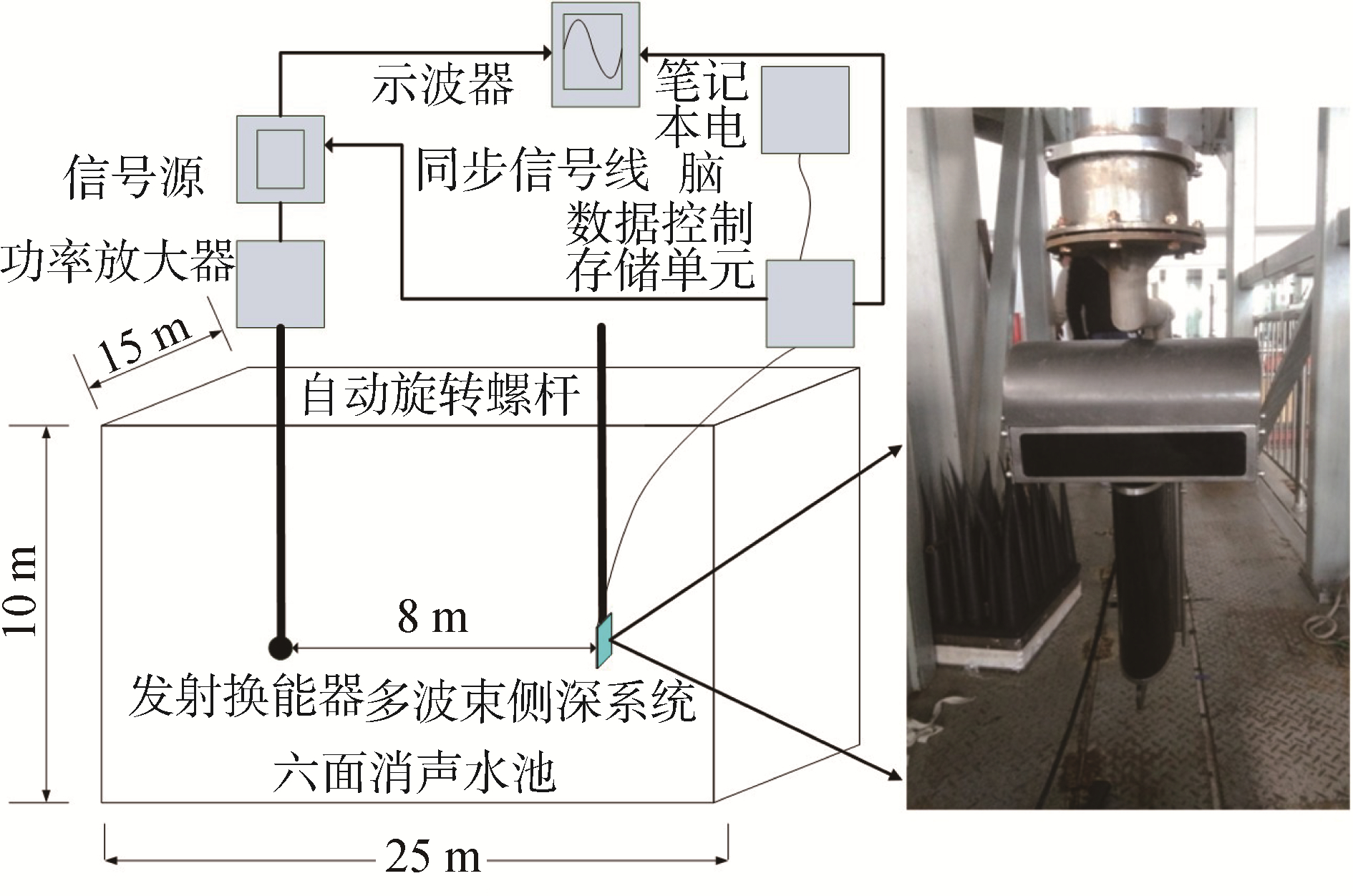Download: 图 6 基阵布放位置示意 Fig. 6 Placement of the transducer array表 2 实验现场工作参数 Table 2 Parameters of the field work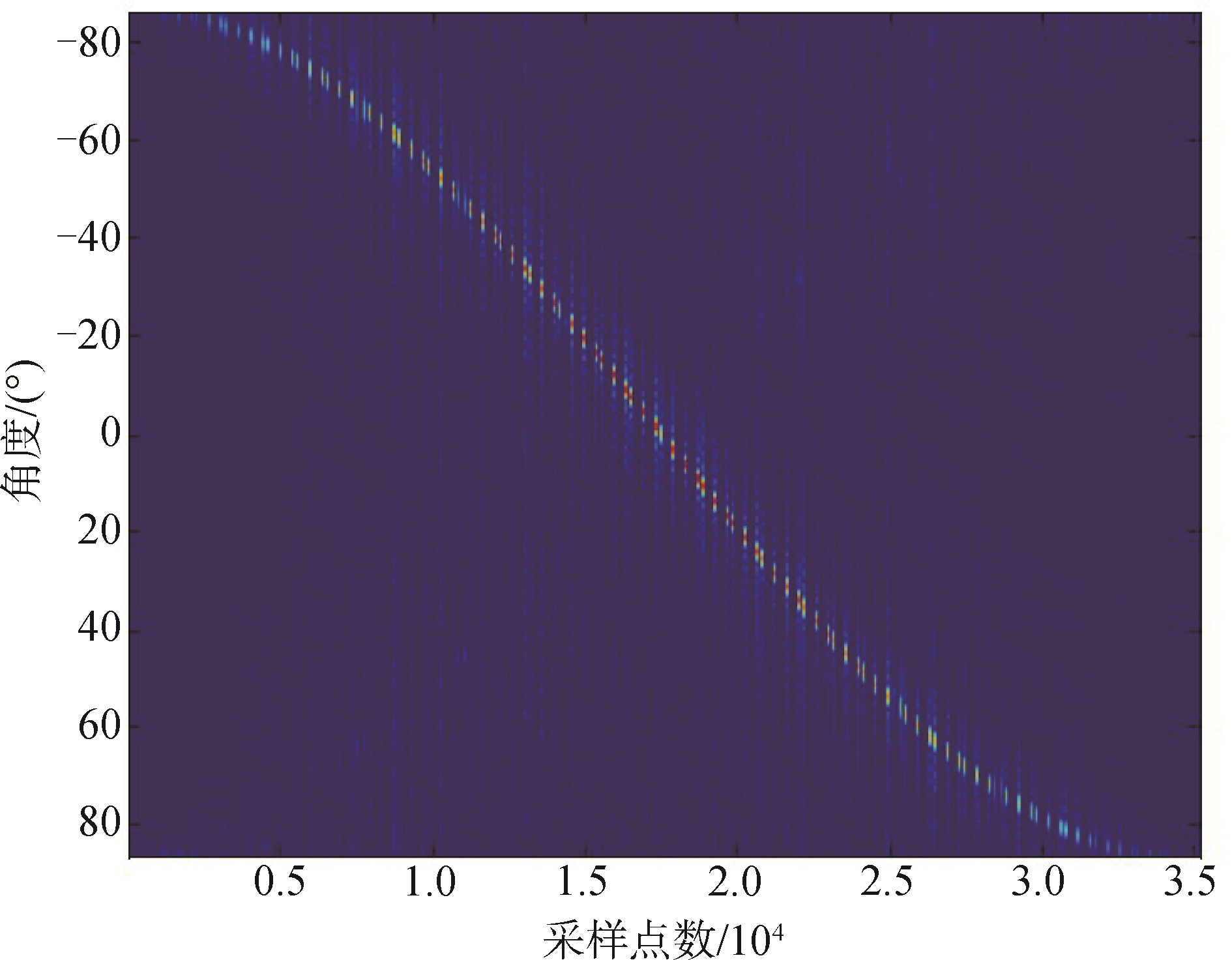Download: 图 7 波束到达方向输出 Fig. 7 Beamforming of the output of DOA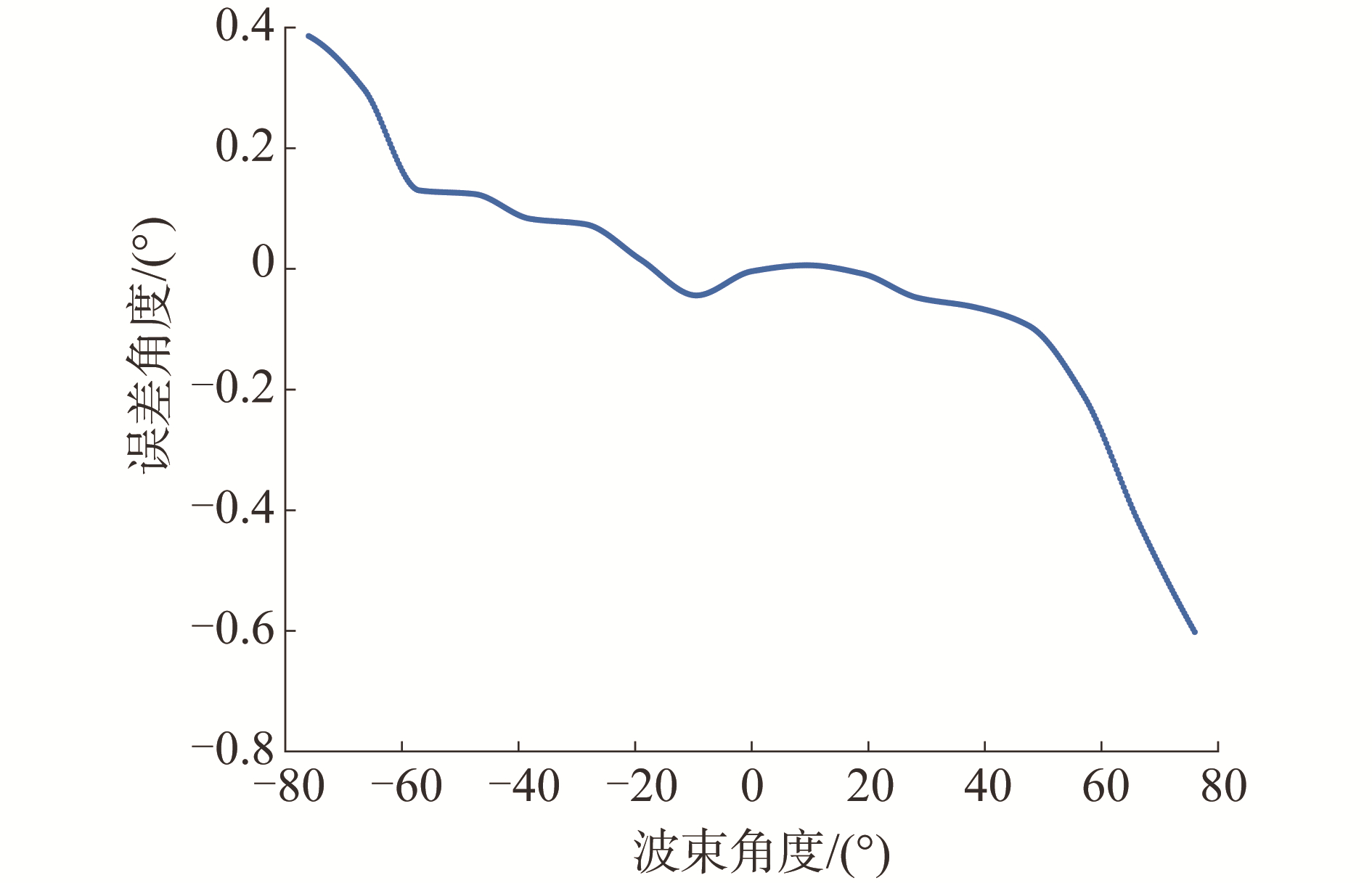Download: 图 8 目标方位波束角误差曲线 Fig. 8 Error curve of the direction of arrival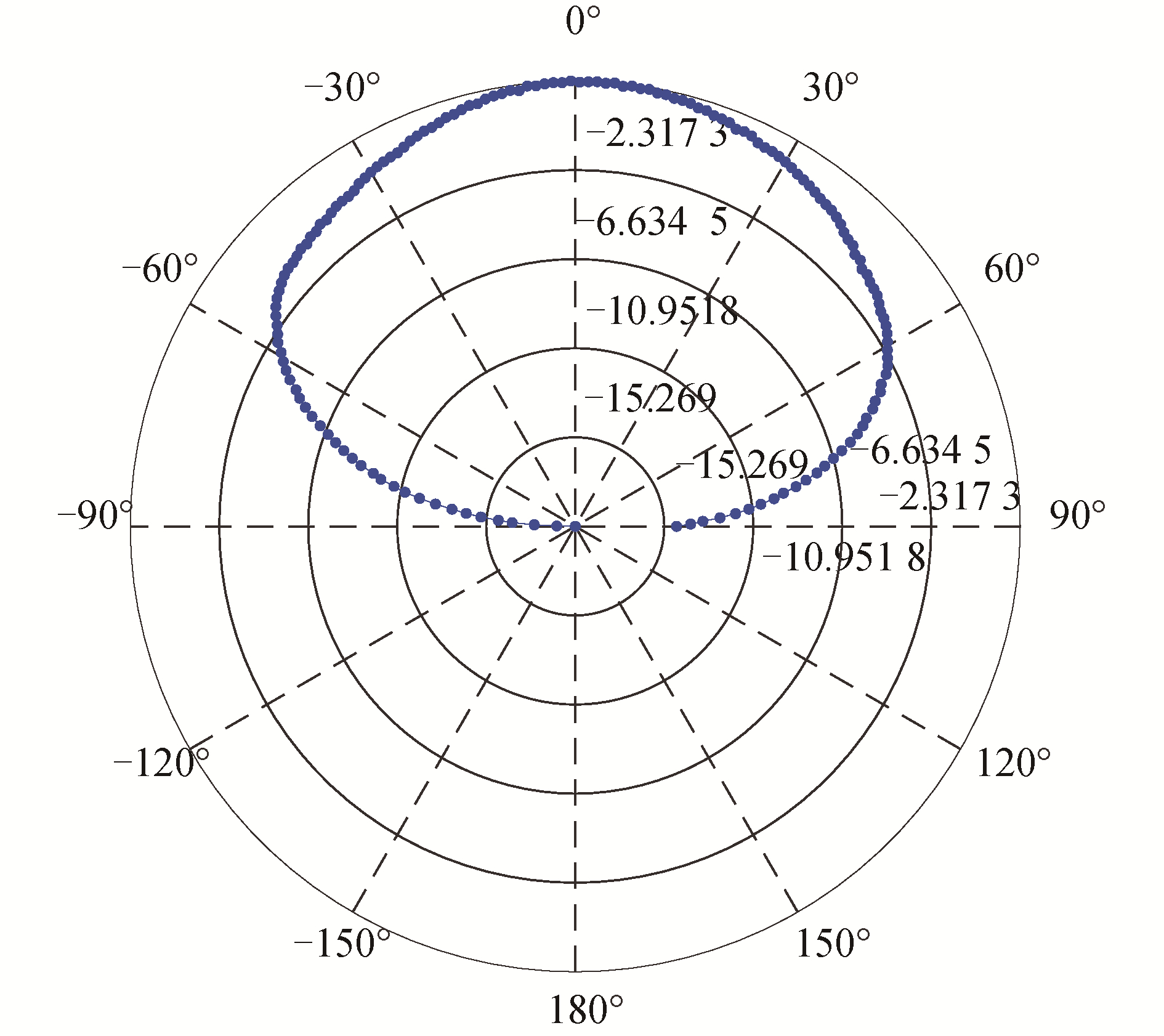Download: 图 9 基阵波束指向性 Fig. 9 Beam directivity of the transducer array
4.2 水池实验测量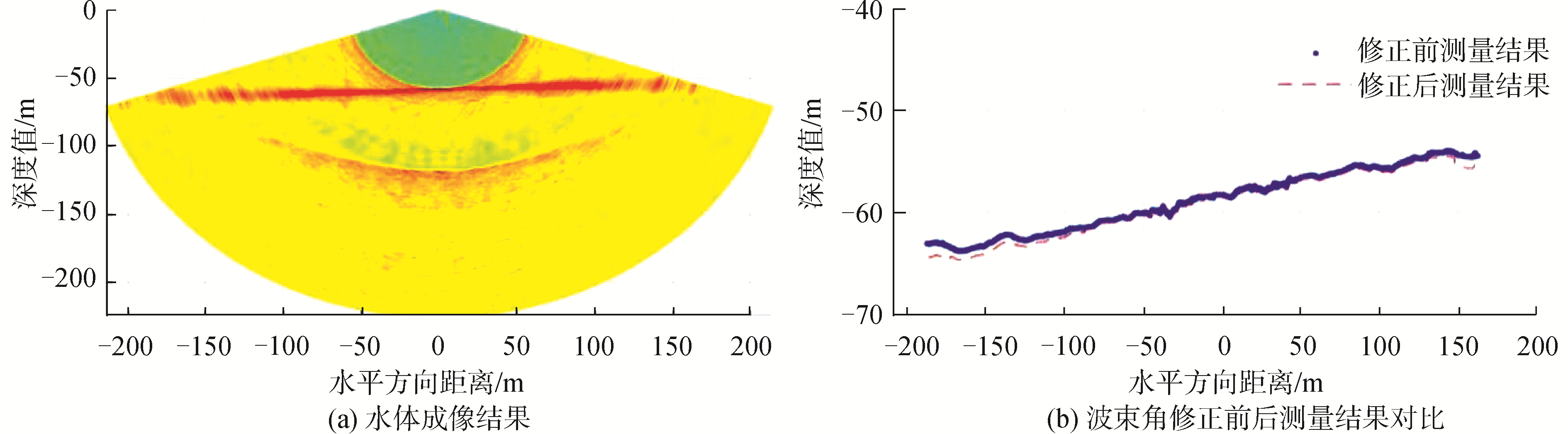Download: 图 10 波束角修正前后水底检测对比 Fig. 10 Comparison of the bottom detection results before and after the beam angle correction

5 结论

  周天, 欧阳永忠, 李海森. 浅水多波束测深声纳关键技术剖析[J]. 海洋测绘, 2016(03): 1-6. ZHOU Tian, OUYANG Yongzhong, LI Haisen. Key technologies of shallow water multibeam bathymetric sonar[J]. Hydro graphic surveying and charting, 2016(03): 1-6. DOI:10.3969/j.issn.1671-3044.2016.03.001 (0)  CHU D, BALDWIN K C, FOOTE K G, Y, et al. Multibeam sonar calibration: target localization in azimuth[C]//Oceans 2001. HI, USA, 2001: 2506-2510. (0)  李海森, 魏波, 杜伟东. 多波束合成孔径声呐技术研究进展[J]. 测绘学报, 2017, 46(10): 1760-1769. LI Haisen, WEI Bo, DU Weidong. Technical progress in research of multibeam synthetic aperture sonar[J]. Cehui Xuebao/Acta Geodaetica et Cartographica Sinica, 2017, 46(10): 1760-1769. DOI:10.11947/j.AGCS.2017.20170410 (0)  XU C, LI H, CHEN B, et al. Angular response classification of multibeam sonar based on multi-angle interval division, Harbin, China, 2016[C]//Institute of Electrical and Electronics Engineers Inc., 2016: 1-4. (0)  LI J, LIN Q, KANG C, et al. DOA estimation for underwater wideband weak targets based on coherent signal subspace and compressed sensing[J]. Sensors (Switzerland), 2018, 18(3): 1-19. (0)  TREES H L V. Detection, estimation, and modulation theory, Part Ⅳ, optimum array processing[J]. A papoulis probability random variables & stochastic processes, 2013, 8(10): 293-303. (0)  尹家亮.多波束测深声呐多通道接收机的设计与实现[D].哈尔滨: 哈尔滨工程大学, 2016. YIN Jialiang. Design and implementation of multi-channel receiver for multi-beam bathymetric sonar[D]. Harbin: Harbin Engineering University, 2016. http://cdmd.cnki.com.cn/Article/CDMD-10217-1017245294.htm (0)  吴自银. 高分辨率海底地形地貌:探测处理理论与技术[M]. 北京: 科学出版社, 2017: 78-80. (0)  孙超. 水下多传感器阵列信号处理[M]. 西安: 西北工业大学出版社, 2007: 51-71. (0)  李海森, 鲁东, 周天. 基于FPGA的多波束实时动态聚焦波束形成方法[J]. 振动与冲击, 2014(03): 83-88. LI Haisen, LU Dong, ZHOU Tian. Multi-beam real-time dynamic focused beam-forming method based on FPGA[J]. Journal of vibration and shock, 2014(03): 83-88. DOI:10.3969/j.issn.1000-3835.2014.03.017 (0)  刘瑞华.基于Sierpinski分形结构的小型宽带振动换能器的设计与制作[D].南京: 南京邮电大学, 2016. LIU Ruihua. Design and fabrication of a miniature broadband vibration transducer based on the sierpinski fractal structure[D]. Nanjing: Nanjing University of Posts and Telecommunications, 2016. http://cdmd.cnki.com.cn/Article/CDMD-10293-1016303288.htm (0)  孙继欣, 张宇, 朱永伟. 压电器件的精密超声复合电解加工技术[J]. 陕西师范大学学报(自然科学版), 2018(05): 32-38. SUN Ji-xin, ZHANG Yu, ZHU Yongwei. Design of precision ultrasonic compound electric maching for piezoelectric components[J]. Journal of Shaanxi Normal University(natural science edition), 2018(05): 32-38. (0)  董玉磊, 桑金. 海道测量规范与IHO标准的比较研究[J]. 海洋测绘, 2018(01): 59-62. DONG Yulei, SANG Jin. Comparative study on specifications for hydrographic survey and the IHO standard[J]. Hydro graphic surveying and charting, 2018(01): 59-62. DOI:10.3969/j.issn.1671-3044.2018.01.015 (0)  MALEIKA W, CZAPIEWSKI P. Visualisation of multibeam echosounder measurement data, Kolkata, India, 2013[C]//Springer Verlag, 2013: 373-380. (0)  KUNTSAL E. A survey of transducer and sonar electroacoustic test and calibration facilities in the USA, 2010[C]//IEEE Computer Society, 2010: 1-6. (0)  薛术.基于2-2型压电复合材料高频线阵换能器的仿真及工艺研究[D].西安: 西安电子科技大学, 2015. XUE Shu. Numerical simulation and process study of a high-frequency linear ultrasonic array based on 2-2 piezoelectric composite[D]. Xi'an: Xi'an Electronic and Science University, 2015. http://www.wanfangdata.com.cn/details/detail.do?_type=degree&id=D01068823 (0)